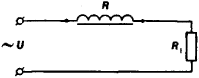# reactor

(redirected from Reactor (disambiguation))
Also found in: Dictionary, Thesaurus.

## reactor

1. Chem a substance, such as a reagent, that undergoes a reaction
2. short for nuclear reactor
3. a vessel, esp one in industrial use, in which a chemical reaction takes place
4. a coil of low resistance and high inductance that introduces reactance into a circuit
5. Med a person sensitive to a particular drug or agent

## Reactor

an induction coil hooked into an electrical circuit to eliminate or suppress the alternating component of current in the circuit or to separate or restrict signals of various frequencies. Reactors are hooked into a circuit in series with a load (see Figure 1): the electrical reactance Z(ω) depends on the frequency of the current:

Z(ω) = ωL

where ω = 2 πf (f is the frequency in hertz) and L is the inductance of the reactor, in henrys. For example, a reactor with high electrical resistance to the alternating component of the current is used as a filter element in current rectifiers.Figure 1. Diagram of connection of a reactor to an electrical circuit: (R) reactor, (R1) load, (U) supply voltage

Reactors are usually made with a core of electrical steel, permalloy, or special materials with high magnetic permeability (such as a ferrite core) to increase inductance, that is, to increase their reactance to alternating current.

## reactor

[rē′ak·tər]
(chemical engineering)
Device or process vessel in which chemical reactions (catalyzed or noncatalyzed) take place during a chemical conversion type of process.
(electricity)
A device that introduces either inductive or capacitive reactance into a circuit, such as a coil or capacitor. Also known as electric reactor.
(nuclear physics)

## Reactor (electricity)

A device for introducing an inductive reactance into a circuit. Inductive reactance x is a function of the product of frequency f and inductance L; thus, x = 2πfL. For this reason, a reactor is also called an inductor. Since a voltage drop across a reactor increases with frequency of applied currents, a reactor is sometimes called a choke. All three terms describe a coil of insulated wire. See Inductor

According to their construction, reactors can be divided into those that employ iron cores and those where no magnetic material is used within the windings. The first type consists of a coil encircling a circuit of iron which usually contains an air gap or a series of air gaps. The air gaps are used to attenuate the effects of saturation of the iron core. The second type, called an air-core reactor, is a simple circular coil, wound around a cylinder constructed of nonmagnetic material for greater mechanical strength. This strength is necessary for the coil to withstand the electromagnetic forces acting on each conductor. These forces become very large with heavy current flow, and their direction tends to compress the coil into less space&colon; radial forces tend to elongate internal conductors in the coil and to compress the external ones while the axial forces press the end sections toward the center of the coil.

Both iron-core and air-core reactors may be of the air-cooled dry type or immersed in oil or a similar cooling fluid. Both types of reactors are normally wound with stranded wire in order to reduce losses due to eddy currents and skin effect. In addition, it is important to avoid formation of short-circuited metal loops when building supporting structures for air-core reactors since these reactors usually produce large magnetic fields external to the coil. If these fields penetrate through closed-loop metal structures, induced currents will flow, causing both losses and heating of the structures. Which of these two reactor types should be used depends on the particular application. See Eddy current

Site: Follow: Share:
Open / Close